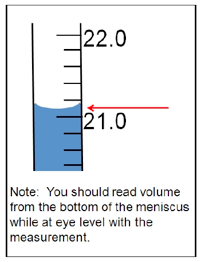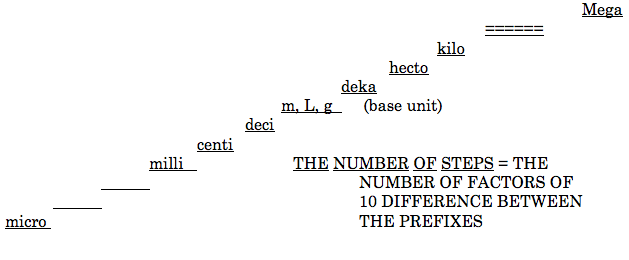## Lab 1 Introduction

### Learning Objectives

By the end of this section, you will be able to:

• Convert numbers from regular notation to scientific notation.
• Perform calculations to the correct number of significant figures.
• Perform calculations using numbers with SI units.
• Convert between base units and units containing prefixes.
• Perform calculations using dimensional analysis.
• Become acclimated with common laboratory equipment

## Introduction

### Scientific Notation

Scientific notation is a way to express numbers. It is especially useful for numbers that are very large or very small. In addition, it uses only significant figures, which is helpful for understanding error (see below). In scientific notation, a number is presented containing two components: a coefficient and the number 10 raised to a power. The coefficient contains a single nonzero number to the left of the decimal
space.

As an example, consider the speed of light: 299,000,000 m/s. We would write this as 2.99 x 108 m/s in scientific notation. The coefficient is 2.99 and must be a number greater than or equal to 1 and less than 10 (one non-zero number will be to the left of the decimal space). The power of 10 is raised to the exponent 8 because you would have to multiply 2.99 by 108 to get the correct number. You can also think about the 8 being from the number of spaces you moved the decimal space. There is an understood decimal at the end of 299,000,000 that we need to move to the right of the number 2 (to allow only a single digit to the left of the decimal). The seven is a positive integer because the number is very large.

Alternatively 0.000003 m is also difficult to express without scientific notation. In order to convert this number to we move the decimal to behind the 3 (the first non-zero number) and add the power of 10 (here we moved the decimal 6 times so the exponent is -6) 3 x 106. Here the exponent is negative because the numb1er is very small (less than 1). Scientific notation is sometimes referred to as exponential notation.

### Significant Figures

Significant figures are an indication of accuracy and precision within a measurement. All calculations in lab should be done to the appropriate number of significant figures. When measuring something in lab, include all known numbers and one estimated number. For example if the meniscus in lab falls halfway between the 21.0 and 21.2 mL marks we know the digits 21 are known. We have to estimate the decimal space because we only know it is larger than 21.0 and smaller than 21.2. Therefore we could say the estimated mark is at the .1 position giving a volume of 21.1 mL. This gives us two numbers we know and one we have estimated or three significant figures. We could not say it was 21.08 mL because that would be two estimated digits instead of one. Numbers are significant if they meet one of the following criteria.• All non-zero numbers are significant. For example, 87 has two significant figures, while 642.45 has five significant figures.
• Zeros sandwiched between two non-zero numbers are significant. Example: 608.5 has four significant figures.
• Leading zeros (zeros at the beginning of very small numbers (numbers < 1) are not significant. For example, 0.038 has two significant figures.
• Trailing zeros are only significant in a number with a decimal. For example 100 has only 1 significant figure. 100.0 has 4 significant figures. 0.01 has one significant figure because there are no trailing zeros after the 1, but 0.010 has 2 significant figures.

### Significant Figures in Calculations

• When multiplying or dividing, your answer should have the same number of significant figures as the number in the calculation with the FEWEST.
• When adding or subtracting, your answer should go to number of decimal spaces as the one in the calculation with the FEWEST number of decimal spaces.

### A Guide to Solving Dimensional Analysis Problems

The following summary can be used as a guide for doing DA. While not all steps listed below will be necessary to solve all problems, any problem can be solved using the following. Do not memorize the sequence of steps, but rather complete practice until you understand how to solve these problems. Dimensional analysis is a fundamental part of chemistry and will be applied all semester. It is imperative you gain an understanding of how to perform calculations using dimensional analysis.

1. Determine what you want to know. Read the problem and identify what you’re being asked to figure out, e.g. “how many milliliters are in 1 liter of solution.”

a. Find starting and ending units:

We are looking for mg in L which means we begin with L and end with mL. You may want to draw out the step(s) you will need for this conversion.

L→mL

2. Determine what you already know.

a. What are you given by the problem, if anything? In this example, we know we have 1 liter of solution.

b. Determine conversion factors that may be needed and write them in a form you can use, such as “60 min/1 hour.”

Here we would need 1 L = 1000 mL Which we can write as a fraction

$\displaystyle\frac{1\text{ L}}{1000\text{ mL}}\\$ or $\displaystyle\frac{1000\text{ mL}}{1\text{ L}}\\$

3. Setup the problem using only what you need to know.

a. Pick a starting factor. If possible, pick what is given, but make sure it is in the appropriate location (top or bottom depending on the final units you want).

b. Set up the problem with all units and making sure conversion factors allow units to cancel. Set up the problem to cancel unwanted units. Our plan is to go to from L→mL using 1 L = 1000 mL

c. If you can’t get to what you want, try picking a different starting factor, or checking for a needed conversion factor.

4. Solve: make sure all the units other than the answer units cancel out, and then do the math.

a. Simplify the numbers by cancellation.
b. Multiply all the top numbers together, then divide into that number all the bottom numbers.
c. Double check to make sure you didn’t press a wrong calculator key by dividing the first top number by the first bottom number, alternating until finished, then comparing the answer to the first one. Miskeying is a significant source of error, so always double check.
d. Round off the calculated answer. Make sure you use the appropriate number of significant figures.

$\displaystyle1\cancel{\text{ L}}\Bigg|\frac{1000\text{ mL}}{1\cancel{\text{ L}}}\Bigg|=1000\text{ mL}\\$

5. Take a few seconds and ask yourself if the answer you came up with makes sense. If it doesn’t, start over.

## Guides

### Significant Figures – Rules

Significant figures are critical when reporting scientific data because they give the reader an idea of how well you could actually measure/report your data. Before looking at a few examples, let’s summarize the rules for significant figures.

1) ALL non-zero numbers (1, 2, 3, 4, 5, 6 ,7, 8, 9) are ALWAYS significant.
2) ALL zeroes between non-zero numbers are ALWAYS significant.
3) ALL zeros which are SIMULTANEOUSLY to the right of the decimal point AND at the end of the number are ALWAYS significant.
4) ALL zeros which are to the left of a written decimal point and are in a number > 1 are ALWAYS significant.

A helpful way to check rules 3 & 4 is to write the number in scientific notation. If you can/must get rid of the zeros, then they are NOT significant.

 Number # Significant Figures Rule(s) 49,923 5 1 3,967 4 1 900.06 5 1, 2, 4 0.004 (=4 E-4) 1 1, 4 8.1000 5 1, 3 501.040 6 1, 2, 3, 4 3,000,000 (=3 E+6) 1 1 10.0 (=1.00 E+1) 3 1, 3, 4

 Prefix Root Abbreviation Decimal (1 with prefix=___ base) Power of Ten mega M 1 000 000 106 kilo k 1 000 103 hecto h 1 00 102 deka da 10 101 METER (base unit) m 1 100 LITER (base unit) L 1 100 GRAM (base unit) g 1 100 deci d 0.1 10-1 centi c 0.01 10-2 milli m 0.001 10-3 micro μ 0.000.001 10-6

##1. Selection modified from: Boundless Scientific Notation 2014 CC-BY-SA 3.0 https://www.boundless.com/chemistry/textbooks/boundless-chemistry-textbook/introduction-to-chemistry- 1/measurement-uncertainty-30/scientific-notation-187-3705/
2. Selection modified from: Boundless Significant Figures 2014 CC-BY-SA 3.0 https://www.boundless.com/chemistry/textbooks/boundless-chemistry-textbook/introduction-to-chemistry- 1/measurement-uncertainty-30/significant-figures-188-7529/
3. Selection modified from: Mrs. Patton 2014 CC-BY-SA Dimensional Analysis with Samples http://math- mrspatton.wikispaces.com/file/links/Dimensional+Analysis+with+Samples.doc